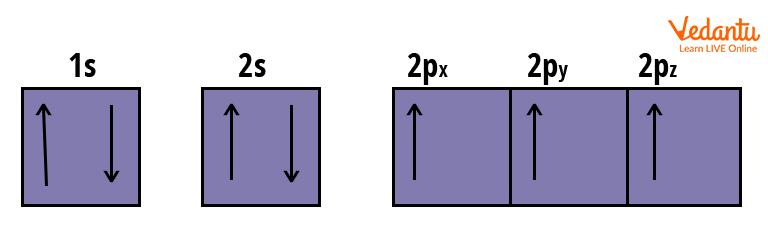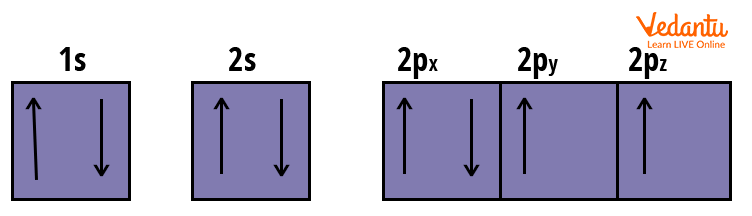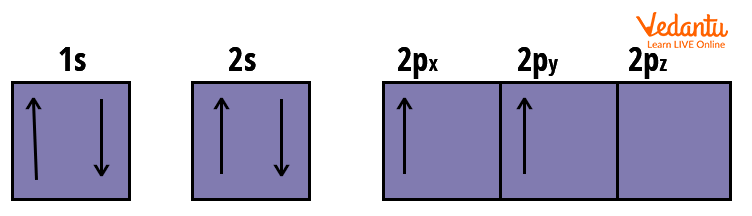Courses
Courses for Kids
Free study material
Free LIVE classes
More

# Hund’s Rule of Maximum Multiplicity for JEE## Introduction

Last updated date: 24th Mar 2023
Total views: 74.1k
Views today: 0.51k

According to Hund’s rule, the lowest energy term for a given electron configuration is the one with the greatest spin multiplicity value. This means that if two or more orbitals of equal energy are available, electrons will occupy them individually before filling them in pairs. Friedrich Hund discovered the rule in 1925 and it is widely used in atomic chemistry, spectroscopy and quantum chemistry. In this article, we state Hund’s rule of maximum multiplicity.

## Hunds Rule of Maximum Multiplicity

What is Hund's rule? Hund's principle states that:

1. Before any orbital in a sublevel is doubly occupied, every orbital is singly occupied.

2. Every electron in a singly occupied orbital has the same spin (to maximise total spin).

Before pairing with another electron in a half-filled orbital, an electron attempts to fill all orbitals with similar energy (also known as ‘degenerate orbitals’). Ground-state atoms have as many unpaired electrons as possible. Consider how electrons behave in this process when visualising it.

As negatively charged electrons fill orbitals, they try to get as far apart as possible before having to pair up, much like how the same poles on a magnet would if they came into contact.

## Rules for Electronic Configuration

The Aufbau principle, Hund's rule and the Pauli Exclusion principle govern the overall electronic configuration of atoms in the periodic table.

• The Aufbau rule dictates that the orbitals with the lowest energy be filled first.

• The Pauli exclusion rule states that no two electrons in the same orbital can have the same spin.

• Hund's rule is concerned with the electronic configuration of orbitals in the same subshell. Here, we explain Hund's rule with example:

p-subshell: Each p-subshell has three orbitals. First, the three orbitals are filled singly. The remaining electrons then enter the orbitals and pair up. They adhere to the Pauli exclusion rule, which states that electrons inside an orbital will have opposite spin.

d-subshell: According to Hund’s rule, the first five electrons in each of the five orbitals have the same spin. Following that, they pair up. When the electron-electron repulsion is minimised, the stability of any electronic configuration increases. Unpaired electrons are the furthest away from each other in the case of a subshell with singly filled degenerate orbitals.

## Examples of Hund’s Rule

Let us examine how Hund's rule influences the electronic configuration of degenerate orbitals in the following atoms:

### Nitrogen Atom

Electronic Configuration: \$1 s^{2}\, 2 s^{2}\, 2 p_{x}^{1}\, 2 p_{y}^{1}\, 2 p_{z}^{1}\$Orbital’s Electronic Arrangement of Nitrogen Atom

The 2p subshell has three electrons in this case. As a result, before pairing, all orbitals will be filled with single electrons.

### Oxygen Atom

Electronic Configuration: \$1 s^{2}\, 2 s^{2}\, 2 p_{x}^{2}\, 2 p_{y}^{1}\, 2 p_{z}^{1}\$Orbital’s Electronic Arrangement of Oxygen Atom

As an oxygen atom has one more electron than a nitrogen atom, the electron in the first orbital of the p-subshell will now pair up.

### Carbon Atom

Electronic configuration : \$1 s^{2}\, 2 s^{2}\, 2 p_{x}^{1}\, 2 p_{y}^{1}\$Orbital’s Electronic Arrangement of Carbon Atom

Carbon, having six electrons, has two electrons paired up each in 1s and 2s orbital and two unpaired electrons in the 2p orbital.

## Applications of Hund’s Rule

• Hund's rule is extremely important in quantum chemistry. It highlights the stability of degenerate orbitals belonging to the same subshell.

• Hund's rule is used in spectroscopy to generate elemental atomic spectra.

• Hund's rule aids in the determination of a stable electronic configuration by ensuring maximum spin multiplicity.

### Purpose of Electron Configurations

The outermost electrons of these atoms or valence shells interact first when they come into contact with one another. When an atom's valence shell isn't full, it's the least stable (thus, the most reactive). The valence electrons of an element heavily influence its chemical behaviour. Chemical characteristics are generally similar across elements with the same number of valence electrons.

Stability can also be predicted using electron configurations. It is the most stable, as all of an atom's orbitals are filled. Later, arrangements are with the highest energy levels and are the most stable. Noble gases have these configurations. These gases are extremely stable substances that do not readily react with other substances. Electron configurations can aid in predicting how particular elements will react, as well as the chemical compounds or molecules that various elements will produce.

## Conclusion

The Aufbau principle, Hund's rule and the Pauli Exclusion principle govern the overall electronic configuration of atoms in the periodic table. The electrons filling the degenerate orbitals achieve the greatest spin multiplicity. This means that when all of the orbitals are filled singly, all the electrons will have the same spin.

The orbital location of an atom's electrons, as well as its overall stability, are determined by its electronic configuration. Hund’s rule electron configuration

has some limitations. It has the drawback of not being able to forecast the lowest energy configuration.

## FAQs on Hund’s Rule of Maximum Multiplicity for JEE

1. What is the multiplicity of spin? Give an example. How many electrons can be available in atomic orbitals of principal quantum number 3 and azimuthal quantum number 2?

The total number of spin orientations represents spin multiplicity. Spin multiplicity is calculated using the formula 2S+1. According to Hund's rule, the highest value of 2S+1 corresponds to the lowest energy or the most stable configuration. Only identical spins result in the maximum value.

The configuration of an orbital is defined by the azimuthal (or orbital angular momentum) quantum number. It is denoted by the symbol 'l' and its value corresponds to the total number of angular nodes in the orbital system. Principal quantum number 3 and azimuthal quantum number 2 denote three-d-orbitals that can hold a maximum of ten electrons.

2. Why do Cu and Cr have exceptional configurations?

Copper and chromium are two of the elements that do not follow the Aufbau principle, which states that electrons are first filled in orbitals of lower energy. This rule is followed by most elements of the periodic table. The reason behind the exceptional configuration of Cu and Cr is the stability of the atom. Half-filled and fully filled electronic configurations are considered extra stable and therefore, copper acquires a full-filled configuration and chromium acquires a half-filled configuration by violating Aufbau’s principle.

3. What is the Pauli exclusion principle and explain its application ?

Wolfgang Pauli was awarded the Nobel Prize in Physics in 1945 for his discovery of electrons. According to the Pauli exclusion principle, no two identical fermions can occupy the same quantum state at the same time. According to the Pauli exclusion principle, each electron must be in its distinct state. In other words, no electron in an atom may have the same set of quantum numbers. The Pauli exclusion principle is central to chemistry, helping to explain electron arrangements in atoms and molecules and rationalising periodic table patterns.